Zou, Y., Wang, Z., Zhang, Y., Zhou, Y., and Shen, Z. (2023). "Cantilever plate mid-span patch method for dynamically testing the in-plane Poisson's ratio of oriented strand board," BioResources 18(1), 1493-1507.

#### Abstract

A cantilever plate span-to-span patch method was proposed and demonstrated for dynamic testing of OSB Poisson’s ratio with high testing accuracy, simplicity, ease of implementation, and effectiveness. It is based on the patch method with zero transverse stress equal to the cantilever plate. Its effectiveness was verified by the four-point bending method and the patch strain gauge method with zero transverse stress. The simulation and theoretical analysis showed that the relative errors of OSB longitudinal and transverse Poisson’s ratios dynamically tested by the span-in patch method and the patch method with zero transverse stress were within ±3.8%. The OSB longitudinal and transverse Poisson’s ratios dynamically tested by the span-in patch method were in good agreement with those tested by the four-point bending method. Thus, the method is applicable to the cantilever plates with l/b= 5 to 6, b/h = 4 to 10, and l/b = 4, b/h = 7 to 10 for cantilevered plates, etc.

Cantilever Plate Mid-Span Patch Method for Dynamically Testing the In-Plane Poisson’s Ratio of Oriented Strand Board

Yuying Zou,a Zheng Wang,a,* Yifan Zhang,b Yuhao Zhou,a and Zhaoyu Shen a

A cantilever plate span-to-span patch method was proposed and demonstrated for dynamic testing of OSB Poisson’s ratio with high testing accuracy, simplicity, ease of implementation, and effectiveness. It is based on the patch method with zero transverse stress equal to the cantilever plate. Its effectiveness was verified by the four-point bending method and the patch strain gauge method with zero transverse stress. The simulation and theoretical analysis showed that the relative errors of OSB longitudinal and transverse Poisson’s ratios dynamically tested by the span-in patch method and the patch method with zero transverse stress were within ±3.8%. The OSB longitudinal and transverse Poisson’s ratios dynamically tested by the span-in patch method were in good agreement with those tested by the four-point bending method. Thus, the method is applicable to the cantilever plates with l/b= 5 to 6, b/h = 4 to 10, and l/b = 4, b/h = 7 to 10 for cantilevered plates, etc.

DOI: 10.15376/biores.18.1.1493-1507

Keywords: Oriented strand board; In-plane Poisson’s ratio; Dynamic testing; Cantilever plate; Mid-span patch method

Contact information: a: Nanjing Forestry University, College of Materials Science and Technology, Nanjing, China, 210000; b: College of Civil Engineering, Southeast University, Nanjing 210037, China; *Corresponding author: wangzheng63258@163.com

INTRODUCTION

Oriented strand board (OSB) is a rapidly developing three-layer solid wood composite that is widely used in furniture, construction, packaging, decoration, and many other fields. It has many advantages including low linear expansion coefficient, high screw holding force, low material consumption, high strength, strong waterproofness, and environmental protection (Tan and Zhou 2006). However, it is challenging to ensure consistency in OSB because of the randomness in the shape, size, and laying orientation of the outer wood chips and small cavities or bubbling on the board surface. The elastic constant of wood and its solid wood composite material is the quantity that characterizes the elasticity of the material (Yi 1996; Wang et al. 2014; Li et al. 2016; Wang 2018; Li et al. 2020; Li et al. 2021; Li et al. 2021; Ponzo et al. 2021). Many researchers have studied the mechanical properties of wood (Ma 1996; Zhou et al. 2007; Cheng and Wang 2015; Wang et al. 2019; Felice et al. 2021), and static and dynamic methods have yielded results in testing its modulus of elasticity, shear modulus, and Poisson’s ratio (Wang et al. 2015; Wang et al. 2016; Zahedi et al. 2020; Li et al. 2021a,b; Tang et al. 2021; Wang and Ghanem 2021; Meng et al. 2021; Zhou et al. 2021). Many research studies used probabilistic methods to study the mechanical properties of materials (Fan and Shen 1992; Thomas 2003; Kumpenza et al. 2018; Peng et al. 2018; Wang and Roger 2021; Wang and Roger 2022). An important material elastic constant required for accurate stress and stiffness analysis of OSB structure is Poisson’s ratio. It has very important engineering value and practical significance to accurately test the Poisson’s ratio of OSB.

The axial tension method is a common method for static testing of Poisson’s ratio of materials. With this method, there are no other special requirements for the position where the strain gauge is glued on the test piece, except for avoiding the vicinity of the chuck of the testing machine or the edge of the test piece. Fan and Shen (1992) used the axial tension method to test the Poisson’s ratio of OSB in the longitudinal and transverse directions and at 45° to the longitudinal direction. Thomas (2003) used the axial tension method to test the principal Poisson’s ratio of OSB. These researchers all tested the Poisson’s ratio of OSB by axial tension method, but the reported values are quite different. Therefore, it is necessary to explore other methods of testing the Poisson’s ratio of OSB. The four-point bending method is another method for statistically testing Poisson’s ratio. To ensure the accuracy of the test, the strain gauge must be attached to the center point of the upper and lower surfaces of the beam. Kumpenza et al. (2018) used the static tension method to test the displacement under load through optical and digital image related technologies and obtained all six main Poisson’s ratios of spruce, but the error of the test value was still large. The dynamic test method for Poisson’s ratio of wood and MDF has been developed (Wang et al. 2015; Gao et al. 2016; Wang et al. 2017; Cao et al. 2019; Wang et al. 2021). To correctly test Poisson’s ratio dynamically, the position of the strain gauge should be located at the position where the internal transverse stress is zero when the cantilever plate undergoes first-order bending vibration. This position is related to the aspect ratio and the width-thickness ratio of the cantilever plate.

This study proposed the cantilever plate mid-span patch method for the dynamic measurement of OSB internal Poisson’s ratio. This method is suitable for cantilever plates with a length-width ratio of 4-6 and a width-thickness ratio of 4-10 as test pieces to dynamically test the longitudinal and transverse Poisson’s ratio (in-plane Poisson’s ratio) of OSB. The cantilever plate mid-span patch method for dynamically testing the in-plane Poisson’s ratio of OSB has a reliable theoretical basis and is quite simple. The validity of the method was verified by the four-point bending method and the glued strain gauge method with zero transverse stress.

EXPERIMENTAL

Cantilever Plate Mid-Span Patch Method

For OSB longitudinal and transverse cantilever plates with aspect ratio l/b=6,5,4,3 and width-thickness ratio b/h=4,7,10, use ANSYS 12.1 modal program is used to calculate the stress and strain of the plate during the first-order bending vibration, and to determine where the transverse stress in the plate equals zero.

Table 1. The Input Parameters of ANSYS Modal Calculation of the Longitudinal and Transverse Blanking Specimens of the Domestic OSB Board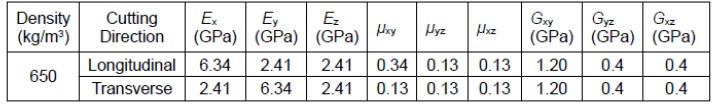The input material parameters calculated by ANSYS are shown in Table 1. Solid45 elements are used to divide the cantilever plate into a grid of 50 × 10 × 3. For the longitudinal and transverse cantilever plates with aspect ratio l/b=6,5,4,3 and width-thickness ratio b/h=4,7,10, a total of 12 positions by x/l where the transverse stress is equal to zero are calculated. After binary linear regression:

When the longitudinal cantilever plate dynamically tests the longitudinal Poisson’s ratio of the OSB, the strain gauge sticking position,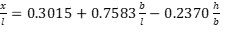(1)

where R equals 0.9867 and n equals 12.

When the transverse cantilever plate dynamically tests the transverse Poisson’s ratio of the OSB, the strain gauge sticking position is as follows: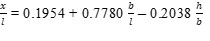(2)

where R equals 0.9950 and n equals 12.

According to equations 1 and 2, when the aspect ratio of the cantilever plate changes from 6 to 3, and the width-thickness ratio changes from 4 to 10, the position x/l where the transverse stress in the plate equals zero, (σy = 0): for the OSB longitudinal plate, x/l changes from 0.38 to 0.53. and for OSB transverse plate, x/l changes from 0.28 to 0.43.

According to the stress of the first-order bending vibration of OSB longitudinal and transverse cantilever plates with aspect ratio l/b=6, 5, 4, 3 and width-thickness ratio b/h=4, 7, 10, the strain calculation result is as follows:

For OSB longitudinal plate and OSB transverse plate, the relative error variation range of the –εy/εx (Poisson’s ratio) of x/l=0.5 (mid-span) and the –εy/εx of x/l determined by the transverse stress equal to zero (σy = 0) are 0.9% to 3% and 0.5% to 1.6%, respectively. In other words, for the cantilever plate with aspect ratio l/b=6 to 3 and width-thickness ratio b/h=4 to 10, it is estimated that the maximum relative error of OSB in-plane Poisson’s ratio will not exceed 3% by using the mid-span patch method to test the value of –εy/εx. For example: for 300 mm × 60 mm × 9.75 mm OSB longitudinal cantilever plate and transverse cantilever plate, the calculated first-order bending stress and strain along the plate length are shown in Figs. 1 and 2, respectively.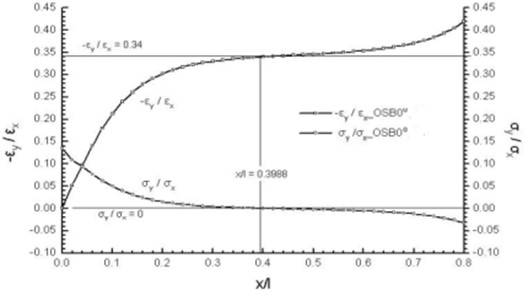Fig. 1.εy/εx vs. x/l and σy/σx vs. x/l curves of OSB longitudinal cantilever plate

Figure shows 1 that the –εy/εx value (Poisson’s ratio) calculated at x/l=0.3988 (σy = 0) differs only by 1.76% from the –εy/εx value calculated at x/l=0.5.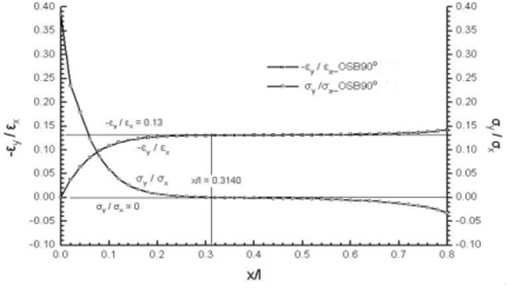Fig. 2.εy/εx vs. x/l and σy/σx vs. x/l curves of OSB longitudinal cantilever plate

Figure 2 shows that the –εy/εx (Poisson’s ratio) calculated at x/l=0.3140 (σy = 0) differs only by 0.77% from the –εy/εx calculated at x/l=0.5. The related icon descriptions are:

OSB0°_-εy/εx represents the distribution curve along x/l of the absolute value of the ratio of the transverse strain to the longitudinal strain of the point on the central line during the first-order bending vibration of the OSB longitudinal cantilever plate.

OSB0°_σy/σx represents the distribution curve along x/l of the ratio of the transverse stress to the longitudinal stress of the point on the central line during the first-order bending vibration of the OSB longitudinal cantilever plate.

OSB90°_-εy/εx represents the distribution curve along x/l of the absolute value of the ratio of the transverse strain to the longitudinal strain of the point on the central line during the first-order bending vibration of the OSB transverse cantilever plate.

OSB90°_σy/σx represents the distribution curve along x/l of the ratio of the transverse stress to the longitudinal stress of the point on the central line during the first-order bending vibration of the OSB transverse cantilever plate.

The main points of the cantilever plate mid-span patch method for dynamic testing of the Poisson’s ratio of OSB are as follows:

1. BX120-10AA strain gauge, the strain gauge size is 10mm×5mm, and the sensitivity coefficient is 2.08.
2. The size requirements of the plate test piece: the clamping depth of the plate is 100 mm. When the ratio of the outer elongation of the plate to the width of the plate l/b=6.5, the ratio of the width to the thickness b/h=4 to 10. When the ratio of the external elongation to the width l/b=4, the ratio of the width to thickness b/h=7 to 10.
3. Cross-shaped strain gauges (middle-span patch) are glued at the center points of the upper and lower plate surfaces of the cantilever plate (extending part of the plate). That is, four strain gauges are attached on the middle span of the cantilever plate. The longitudinal strain gauges and transverse strain gauges on the upper and lower plates are respectively connected by the half-bridge method.
4. The in-plane Poisson’s ratio μxy of OSB is measured for the cantilever plate longitudinal specimen cut along the whole OSB plate longitudinal (X direction), and the in-plane Poisson’s ratio μyx of OSB is measured for the cantilever plate transverse specimen cut along the whole OSB plate transverse (Y direction).
5. The knocking point is on the center line of the plate near the free edge to excite the free vibration of the cantilever plate. Linear spectral amplitude value of the transverse strain and longitudinal strain are taken at the first-order bending frequency of the spectrum.
6. Poisson’s ratio is defined as the ratio of the linear spectral amplitude of the transverse strain to the linear spectral amplitude of the longitudinal strain at the first-order bending frequency on the cantilever plate spectrogram, %, namely: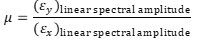(3)

Dynamic Test of OSB’s In-Plane Poisson’s Ratio

The longitudinal and transverse OSB specimens were prepared from a whole Canadian OSB board along the longitudinal and transverse directions, respectively, as shown in Fig. 3. The basic parameters of the test piece are shown in Table 2.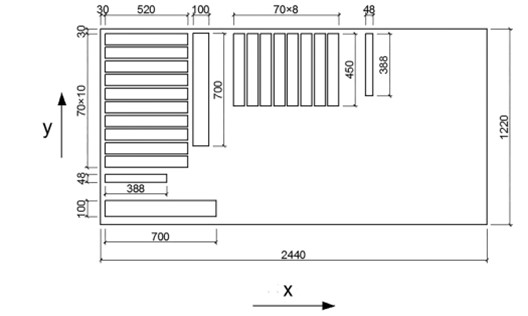Fig. 3. Schematic diagram of cutting the longitudinal and transverse specimens of OSB made in Canada (mm)

Table 2. Basic Parameters of OSB Longitudinal and Transverse Specimens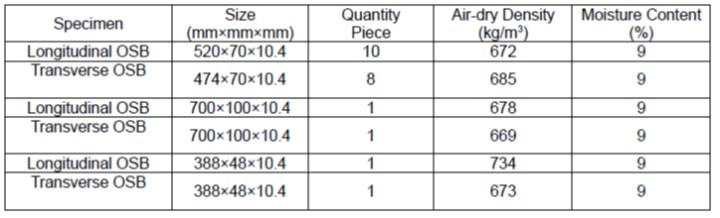Test Equipment

The test uses 1 set of CRAS vibration and dynamic signal acquisition and analysis system, including signal conditioning instrument, AZ acquisition box and its supporting analysis software. There is a YD-28A dynamic strain gauge and a BX120-10AA strain gauge (sensitivity coefficient 2.08, strain grid length 10 mm × width 5 mm). In addition there is one set of cantilever plate holding devices, one set of four-point bending test devices, one force hammer, several weights, and several wires.

Experiment Design for Dynamic Testing of OSB’s Longitudinal Poisson’s Ratio

(1) The size of the OSB longitudinal specimen was 520 mm × 70 mm × 10.4 mm, with a total of 10 pieces. The clamping depth of the cantilever plate was 100 mm, the outer elongation was 420 mm, and the longitudinal and transverse strain gauges were glued on the center line of the upper and lower plate surfaces 210 mm away from the fixed end. The longitudinal and transverse strain gauges respectively occupied the two channels of the strain gauge according to the half-bridge method to ensure that the cantilever plate with l/b=6 and b/h=6.7. Dynamic testing was done for the ratio of the linear spectrum amplitude of the transverse strain to the longitudinal strain at the first-order bending frequency.

(2) A cantilever plate with an external elongation of 358 mm (l/b=5.11) was obtained. Determined by the position where (σy = 0), and referring to formula (1), the position of the strain gauge was at x/l=0.413. A dynamic test was carried out for the ratio of the linear spectrum amplitude of the transverse strain to the longitudinal strain at the first-order bending frequency.

(3) The specimen was sawn so that the distance between the pasted strain gauge and the free end of the cantilever plate was 175 mm, and the external elongation was 350 mm. In this case, the strain gauge was located in the middle of the cantilever plate, and the cantilever plate with l/b=5, b/h=6.7 was obtained. The ratio of the linear spectrum amplitude of the transverse strain to the longitudinal strain at the first-order bending frequency was dynamically tested.

(4) The next step was to change the clamping depth to achieve a cantilever plate with an external elongation of 310 mm (l/b=4.43). In this case, the position of the pasted strain gauge was at x/l=0.436, which was determined by Eq. 1, and the ratio of the linear spectrum amplitude of the transverse strain to the longitudinal strain at the first-order bending frequency was dynamically tested.

(5) The specimen was sawn so that the glued strain gauge was 140 mm away from the free end of the cantilever plate to achieve an external elongation of 280 mm cantilever plate. In this case, the strain gauge was placed in the middle of the cantilever plate, and the cantilever plate with l/b=4, b/h=6.7 was obtained. The ratio of the linear spectrum amplitude of the transverse strain to the longitudinal strain at the first-order bending frequency was dynamically tested.

(6) The next step was to change the clamping depth to achieve a cantilever plate with an external elongation of 264 mm (l/b=3.77). At this time, the position of the pasted strain gauge was x/l=0.468, as determined by Eq. 1, and the ratio of the linear spectrum amplitude of the transverse strain to the longitudinal strain at the first-order bending frequency was dynamically tested.

Experiment Design for Dynamic Testing of OSB’s Transverse Poisson’s Ratio

(1) The size of transverse specimen was 450 mm × 70 mm × 10.4 mm, with a total of 8 pieces. The clamping depth of the cantilever plate was 100 mm, and the outer elongation was 350 mm. Longitudinal and transverse strain gauges were attached on the center line of the upper and lower plate surfaces 175 mm away from the fixed end. The longitudinal and transverse strain gauges, respectively, occupied the two channels of the strain gauge according to the half-bridge method to obtain the cantilever plate with l/b=5 and b/h=6.7. Dynamic testing was done for the ratio of the linear spectrum amplitude of the transverse strain to the longitudinal strain at the first-order bending frequency.

(2) A transverse cantilever plate with an external elongation of 275 mm (l/b=3.93) is obtained. Determined by the position where (σy = 0), and referring to formula (2), the position of the strain gauge was at x/l=0.363. The ratio of the linear spectrum amplitude of the transverse strain to the longitudinal strain was dynamically tested at the first-order bending frequency.

(3) The specimen was sawn so that the distance between the pasted strain gauge and the free end of the transverse cantilever plate was 140 mm, and the external elongation was 280 mm. In this case, the strain gauge was located in the middle of the cantilever plate, and the cantilever plate with l/b=4, b/h=6.7 was obtained. The ratio of the linear spectrum amplitude of the transverse strain to the longitudinal strain at the first-order bending frequency was dynamically tested.

(4) The last step was to change the clamping depth to achieve a transverse cantilever plate with an external elongation of 233 mm (l/b=3.33). In this case, the position of the pasted strain gauge was at x/l=0.3985, which is determined by Eq. 2, and the ratio of the linear spectrum amplitude of the transverse strain to the longitudinal strain at the first-order bending frequency was dynamically tested.

Dynamically Test the Longitudinal and Transverse Poisson’s Ratio of OSB

The OSB longitudinal and transverse specimens were 700 mm × 100 mm × 10.4 mm each, and the clamping depth was 100 mm. The cantilever plates with aspect ratios of l/b=6, 5, and 4 were obtained by sawing, and their width-thickness ratio were all 9.6. The longitudinal and transverse specimens of OSB were 388 mm × 48 mm × 10.4 mm, 1 piece each, and the clamping depth was 100 mm. For the cantilever plate with the aspect ratio l/b of 6, 5 and 4, respectively, and the width-thickness ratio b/h was 4.6. The longitudinal and transverse Poisson’s ratios of OSB were measured by clamping specimens at the position where the strain gauge was located in the middle of the span and the position where the transverse stress is 0, which means that σy = 0.

Dynamic Test Principle and Test Block Diagram

The half-bridge method of longitudinal strain gauges on the upper and lower surfaces of the cantilever plate occupies one channel of the dynamic strain gauge, and the half-bridge method of the transverse strain gauges on the upper and lower surfaces of the cantilever plate occupies another channel of the dynamic strain gauge, which is dual-channel measurement. The output of the strain gauge is amplified and filtered by a signal conditioner, and the filter frequency is set to only the first-order frequency of the cantilever plate on the spectrogram. The output signal of the signal conditioner is converted from an analog signal to a digital signal through a signal acquisition box, and then signal analysis software and computer calculations show the spectrum of the cantilever plate. The test diagram is shown in Fig. 4.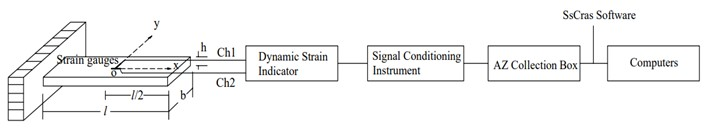Fig. 4. Experimental block diagram of dynamic test of OSB longitudinal and transverse Poisson’s ratio

Four-point Bending Test-Confirmatory Test

A static four-point bending test was used for verification, as shown in Fig. 5. The four-point bending test piece was sawn from the test piece of the cantilever plate mid-span patch to test the longitudinal and transverse Poisson’s ratio of the OSB. The size of the specimen was 280 mm × 28 mm × 10.4 mm, and the span of the specimen was l=240 mm. The longitudinal and transverse strain gauges were located in the middle of the specimen, and the longitudinal and transverse strain gauges of the upper and lower plates were measured according to the half-bridge method. There were 10 longitudinal specimens and 8 transverse specimens. Poisson’s ratio was calculated according to Eq. 3,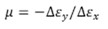(3)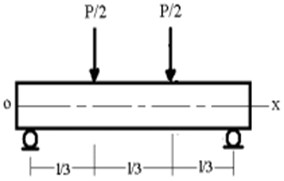RESULTS AND DISCUSSION

Figures 6 and 7 show the strain spectrograms of the longitudinal No. 6 and transverse.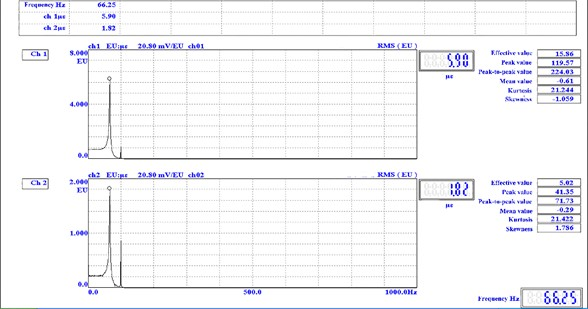Fig. 6. The frequency spectrum of the longitudinal cantilever plate specimen (280 mm×70 mm×10.4 mm)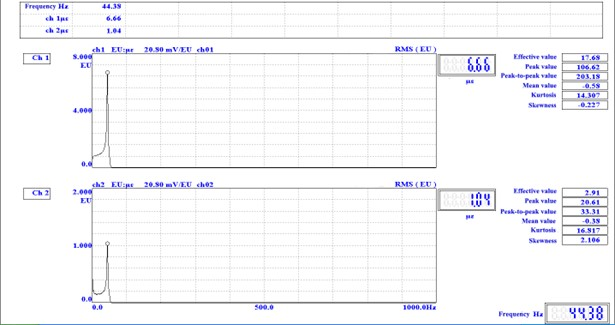Fig. 7. The frequency spectrum of the horizontal cantilever plate specimen (280mm×70mm×10.4mm)

No. 1 cantilever plate specimens tested by the mid-span patch method, respectively, from which the transverse strain linear spectrum amplitude and longitudinal strain linear spectrum amplitude at the first bending frequency can be obtained. Figure 6 shows that the Poisson’s ratio calculated from the linear spectrum amplitude of the longitudinal strain and the transverse strain at the first-order bending frequency of 66.25 Hz was 1.82/5.9=0.307.

Figure shows 7 that the Poisson’s ratio calculated from the linear spectrum amplitude of the longitudinal strain and the transverse strain at the first-order bending frequency of 44.38Hz was 1.04/6.66=0.156.

When longitudinal and transverse OSB specimens and with different aspect ratios were patched in the middle of the span, the results of the longitudinal and transverse Poisson’s ratios of the OSB obtained by the dynamic test and four-point bending test were as shown in Table 3 (the percentage in parentheses is the coefficient of variation).

Table 3. OSB’s Longitudinal and Transverse Poisson’s Ratios Tested by the Mid-Span Patch Method and the Four-Point Bending Method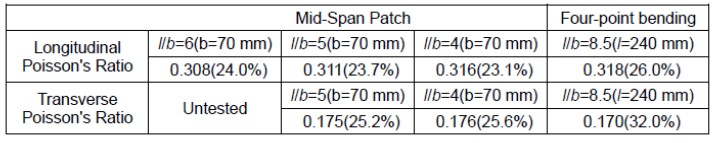Tables 4 and 5 show the longitudinal Poisson’s ratio and transverse Poisson’s ratio of the OSB tested by the mid-span patch method and the transverse stress equal to zero (σy = 0) patch method, respectively.

Table 4. OSB’s Longitudinal Poisson’s Ratio of the Dynamic Test of the Mid-span Patch Method and the Transverse Stress (σy = 0) Patch Method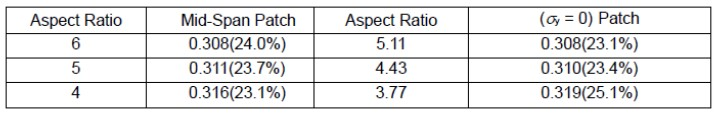Table 5. OSB’s Transverse Poisson’s Ratio of the Dynamic Test of the Mid-span Patch Method and the Transverse Stress (σy = 0) Patch Method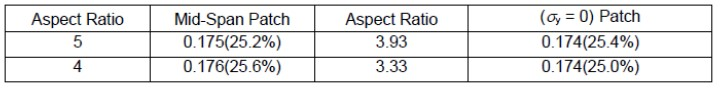There are 12 test conditions for the longitudinal and transverse cantilever plates when l/b=6, 5, 4, b/h=9.6 and l/b=6, 5, 4, b/h=4.6. According to the measured data in Table 5, it can be seen that the relative error of OSB transverse Poisson’s ratio measured by the mid-span patch method and the transverse stress equal to zero (σy = 0) patch method was as large as 11.9% only in a test condition when the l/b=4 and the b/h=4.6 for transverse cantilever plate. For the other 11 test conditions, the relative error of the OSB’s longitudinal or transverse Poisson’s ratio test values of the mid-span patch method and the transverse stress equal to zero (σy = 0) patch method was within ±3.8%, as shown in Table 6.

Table 6. Dynamic Test Values of OSB’s Longitudinal and Transverse Poisson’s Ratio of the Mid-Span Patch Method and the Transverse Stress (σy = 0) Patch Method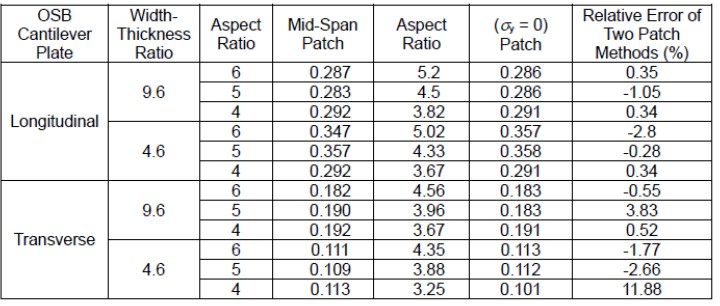Analysis of Test Results

(1) The maximum relative errors of the longitudinal and transverse Poisson’s ratios of OSB tested by the mid-span patch method and the four-point bending method were -3.1% and +3.5%, respectively, as shown in Table 3.

(2) When the aspect ratio of the cantilever plate was l/b=6, 5, 4, and the width-thickness ratio was b/h=6.7, the relative error of the longitudinal or transverse Poisson’s ratio of the OSB dynamically tested by the mid-span patch method and (σy = 0) patch method was within ± 2%, as shown in Tables 4 and 5. The test results were in good agreement with the results of ANSYS calculation examples, as shown in Figs. 1 and 2.

(3) When the aspect ratio of the cantilever plate l/b was 6, 5, 4, and the width-thickness ratio b/h was 4.6, 9.6, the relative error of the longitudinal or transverse Poisson’s ratio of OSB obtained by the mid-span patch method and (σy = 0) patch method dynamic test was within ±3.8%, as shown in Table 6, (except for the transverse Poisson’s ratio data of the transverse cantilever plate with l/b=4 and b/h=4.6).

(4) The mid-span patch method for dynamic testing of OSB’s longitudinal and transverse Poisson’s ratio is suitable for l/b=6-5, b/h=4-10, and l/b=4, b/h=7-10. The accuracy of the OSB’s longitudinal and transverse Poisson’s ratio can be guaranteed for the cantilever plate with the aspect ratio l/b and the width-thickness ratio b/h that meets the above requirements, as shown in Tables 3 to 6.

Theory Analysis

Considering the anisotropy of OSB, the stress-strain relationship of the plate (on the xy coordinate plane) can be expressed as: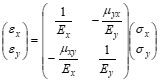(4)

In formula 4, μxy represents the absolute value of the ratio of y-direction strain εy to x-direction strain εx under the action of unidirectional stress σx, which means that μxy equals –εy/εx. The quantity μxy represents the absolute value of the ratio of x-direction strain εx to y-direction strain εy under the action of unidirectional stress, which means that μyx equals –εx/εy.

According to the symmetry of the flexibility matrix, one may state that –μxy/Ex = –μyx/Ey, which means that Eyμxy = Exμyx. If σy = 0, then by formula (4), μxy = –εy/εx. When the strain gauge is attached to the σy = 0 position, the absolute value of the ratio of the measured value of the transverse strain to the longitudinal strain is equal to the Poisson’s ratio. If σy does not equal 0, then –εy/εx = [(Ex/Ey)(σy /σx) –μxy]/[1-μyx (Ex/Ey)(σy /σx)] is obtained by formula (4).

According to the symmetry of the flexibility matrix: Eyμxy = Exμyx, the result is: εy/εx = [(Ex/Ey)(σy /σx) –μxy]/[1-μyx (Ex/Ey)(σy /σx)].

According to the calculation results of ANSYS, for the longitudinal and transverse cantilever plates with aspect ratio of 5-6, width-thickness ratio of 4-10, and aspect ratio of 4, width-thickness ratio of 7 to 10, at the position of x/l=0.5, which is the mid-span of the cantilever plate, the dynamic stress of its first-order bending vibration σy/σx < -0.005, and the ratio of the longitudinal and transverse elastic modulus of OSB Ex/Ey < 3. Therefore, when the cantilever plate reaches the first-order bending vibration, the ratio of the transverse strain to the longitudinal strain εy/εx and the Poisson’s ratio μxy in the middle of the span can be approximated as μxy ≅ -(εy/εx) + (Ex/Ey)(σy /σx); μxy ≅ -(εy/εx) + 0.15, which means that the ratio of the transverse and longitudinal strain test values in the middle of the OSB cantilever plate εy/εx has sufficient accuracy to characterize the Poisson’s ratio.

In summary, the cantilever plate mid-span patch method for dynamic testing of OSB’s longitudinal and transverse Poisson’s ratio has a reliable theoretical basis, and has been verified by the tests of the lateral stress equals to zero patch method and the four-point bending method. The cantilever plate mid-span patch method is effective in testing the longitudinal and transverse Poisson’s ratio of OSB. For cantilever plates with l/b=6-5, b/h=4-10 and l/b=4, b/h=7-10, the accuracy of the mid-span patch method in testing the OSB’s longitudinal and transverse Poisson’s ratio is ensured sufficient accuracy. Therefore, the mid-span patch method is a simple and easy way to dynamically test the longitudinal and lateral Poisson’s ratio of OSB.

CONCLUSIONS

• The cantilever plate mid-span patch method for dynamic testing of oriented strand board’s (OSB’s) longitudinal and lateral Poisson’s ratio based on the patch method where the transverse stress (σy = 0) has a reliable theoretical basis.
• From simulation calculations, theoretical analysis, and experiments, it is shown that the use of the cantilever plate mid-span patch method to dynamically test the longitudinal and transverse Poisson’s ratio of OSB has sufficient accuracy. At the same time, this method is a simple, easy, and effective way to dynamically test the longitudinal and transverse Poisson’s ratio of OSB.
• The cantilever plate mid-span patch method for dynamic testing of OSB’s longitudinal and transverse Poisson’s ratio is suitable for the cantilever plate with aspect ratio l/b=5 to 6, width-thickness ratio b/h=4 to 10, and aspect ratio l/b= 4, width-thickness ratio b/h=7 to 10.

ACKNOWLEDGMENTS

This work was supported by 2021 Jiangsu Province Agricultural Science and Technology Independent Innovation Fund project [CX(21)3049].

REFERENCES CITED

Cao, Y., Li, M. M., W Z, and Wang, Y. L. (2019). “Dynamic testing and analysis of Poisson’s ratio of lumbers based on the cantilever-plate bending mode shape method.” Journal of Testing and Evaluation 47(4), 2540-2550. DOI: 10.1520/JTE20160521

Cheng, K., and Wang, Z. (2015). “New method for testing shear modulus based on torsional vibration shape of free plate,” Journal of Nanjing Tech University (Natural Science Edition) 37(5), 61-66. DOI: 10.3969/j.issn.1671-7627.2015.05.010.

Fan, W. Y., and Shen, F. M. (1992). “Test of elastic modulus and Poisson’s ratio of oriented strand board,” China Forest Science and Technology 15(3), 39-40. DOI: 10.13360/j.issn.1000-8101.1992.03.022.

Gao, Z. Z., Zhang, X., Wang, Y. L., Yang, R. C., Wang, G. G., and Wang, Z. (2016). “Measurement of the Poisson’s ratio of materials based on the bending mode of the cantilever plate,” BioResources 11(3), 5703-5721. DOI: 10.15376/biores.11.3.5703-5721.

Kumpenza, C., Matz, P., Halbauer, P., Grabner, M., Steiner, G., Feist, F., and Müller, U. (2018). “Measuring Poisson’s ratio: Mechanical characterization of spruce wood by means of non-contact optical gauging techniques,” Wood Science and Technology 52, 1451-1471. DOI: 10.1007/s00226-018-1045-7.

Li, H. T., Zhang, Q. S., and Wu, G. (2016). “A review on development of laminated bamboo limber,” Journal of Forestry Engineering 1(6), 110-116. DOI: 10.13360/j.issn.2096-1359.2016.06.002

Li, H. T., Xuan, Y. W., and Xu, B. (2020). “Bamboo application in civil engineering fields,” Journal of Forestry Engineering 5(6), 1-10. DOI: 10.13360/j.issn.2096-1359.202003001.

Li, H. T., Wang, B. J., Wang, L. B., Wei, P. X., Wei, Y., and Wang, P. Z. (2021a). “Characterizing engineering performance of bamboo-wood composite cross-laminated timber made from bamboo mat-curtain panel and hem-fir lumber.” Composite Structures 266, article 113785.

Li, H. T., Wang, L. B., Wang, B. J., Wei, P. X., Yu, W. T., Fan, Z. Q., and Du, G. B. (2021b). “Preliminary evaluation of a density-based lumber grading method for hem-fir CLT manufacturing,” European Journal of Wood and Wood Products 79(4)967-975.

Li, H., Wang, L. B., Wei, Y., Wang, B. J., and Jin, H. (2022a). “Bending and shear performance of cross-laminated timber and glued-laminated timber beams: A comparative investigation,” Journal of Building Engineering 45, article 103477.

Li, H., Wang, L. B., Wei, Y., and Wang, B. J. (2022b). “Off-axis compressive behavior of cross-laminated bamboo and timber wall elements,” Structures 35, 452-468.

Ma, G. X. (1996). “Dynamic (static) measurement of the elastic constants in uniaxially-reinforced composite sheet,” Acta Materiae Compositae Sinica 13(2), 117-123. DOI: 10.13801/j.cnki.fhclxb

Meng, Z., Zhang, D., Feng, P., and Hu, N. (2021). “Review on mechanical behavior of solar cells for building integrated photovoltaics,” Sustainable Structures 1(2), article 000009. DOI: 10.54113/j.sust.2021.000009

Peng, Y. B., Wang, Z. H., and Ai, X. Q. (2018). “Wind-induced fragility assessment of urban trees with structural uncertainties,” Wind & Structures 26(1), 45-56.

Ponzo, F. C., Antonio, D. C., Nicla, L., and Nigro, D. (2021). “Experimental estimation of energy dissipated by multistorey post-tensioned timber framed buildings with anti-seismic dissipative devices,” Sustainable Structures 1(2), article 000007.

Tan, S. X., and Zhou, D. G. (2006). Handbook of Wood Industry, Forestry Publishing House, Beijing, China, pp. 261-306.

Tang, M., Liu, Y., Ding, F., and Wang, Z. (2021). “Solution to solid wood board cutting stock problem,” Applied Sciences 11(17), article 7790.

Thomas, W. H. (2003). “Poisson’s ratios of an oriented strand board,” Wood Science and Technology 37, 259-268. DOI: 10.1007/s00226-003-0171-y

Wang, Z., Wang, Z., Wang, B. J., Wang, Y., Rao, X., Liu, B., Wei, P., and Yang, Y. (2014). “Dynamic testing and evaluation of modulus of elasticity (MOE) of SPF dimension lumber,” BioResources 9(3), 3869-3882.

Wang, Z., Gao, Z., Wang, Y., Cao, Y., Wang, G., Liu, B., and Wang, Z. (2015). “A new dynamic testing method for elastic, shear modulus and Poisson’s ratio of concrete,” Construction and Building Materials 100, 129-135.

Wang, Z., Gu, L. L., Gao, Z. Z., Liu, B., and Wang, Y. L. (2015). “Experimental study on Poisson’s ratio of lumber by dynamic testing,” Scientia Silvae Sinicae 51(5), 102-107. DOI: 10.11707/j.1001-7488.20150512

Wang, Z., Wang, Y., Cao, Y., and Wang, Z. (2016). “Measurement of shear modulus of materials based on the torsional mode of cantilever plate,” Construction and Building Materials 124, 1059-1071.

Wang, Y. L., Wang, Z., Li, M. M., and Cao, Y. (2017). “Discussion on static testing method of material MDF constants of elastic modulus, Passion’s ratio and shear modulus,” Journal of Beijing Forestry University 39(10), 117-121. DOI: 10.13332/j.1000-1522.20170107

Wang, Z., and Ghanem, R. (2021). “An extended polynomial chaos expansion for PDF characterization and variation with aleatory and epistemic uncertainties,” Computer Methods in Applied Mechanics and Engineering 382, article 113854.

Wang, Z., and Ghanem, R. (2022). “A functional global sensitivity measure and efficient reliability sensitivity analysis with respect to statistical parameters,” Computer Methods in Applied Mechanics and Engineering, 115175.

Wang, Z., Xie, W. B., Lu, Y., Li, H., and Wang, Z. (2019). “Dynamic and static testing methods for shear modulus of oriented strand board,” Construction and Building Materials 216, 542-551.

Wang, Z., Xie, W., Wang, Z., and Cao, Y. (2018). “Strain method for synchronous dynamic measurement of elastic, shear modulus and Poisson’s ratio of wood and wood composites,” Construction & Building Materials 182, 608-619.

Wang, Z. G., Zhuang, Z. L., Liu, Y., Ding, F. L., and Tang, M. (2021). “Color classification and texture recognition system of solid wood panels,” Forests 12(09), 1154.

Yi, S. C. (1996). Wood Science, China Forestry Publishing House, China, pp. 160-163.

Zahedi, M., Kazemi, S., Füssl, J., and Elyasi, M. (2020). “Characterization of engineering elastic parameters of oriented strand board (OSB) manufactured from poplar (Populus deltoides) strands using ultrasonic contact pulse transmission,” Drvna Industrjia 71(3), 227-234. DOI: 10.5552/drvind.2020.1908.

Zhou, H. B., Ren, H. Q., and Fei, B. (2007). “Dynamical test on flexural and shear modulus of composite wood panels,” Journal of Building Materials 10(5), 561-565. DOI: 10.1016/S1872-5791(07)60033-5

Zhou, Y., Huang, Y., Sayed, U., and Wang, Z. (2021). “Research on dynamic characteristics test of wooden floor structure for gymnasium,” Sustainable Structures 1(1), Article No. 000005. DOI: 10.54113/j.sust.2021.000005

Article submitted: August 3, 2022; Peer review completed: November 5, 2022; Revised version received: November 22, 2022; Accepted: November 28, 2022; Published: January 9, 2023.

DOI: 10.15376/biores.18.1.1493-1507